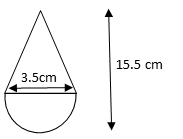# CBSE Class X Board Solution

2017 Math Set 3 - Q18. Surface Area

#### Video ExplanationTotal height of toy = 15.5 cm
Radius of hemisphere = 3.5cm = $$frac{7}{2}$ cm Therefore, height of conical portion of toy = 12 cm Surface area of toy = lateral surface area of cone + surface area of hemisphere = πrl + 2πr2, where ‘r’ is the radius$cone and hemisphere have the same radii) and ‘l’ the slant height of the cone.

Slant height of the cone, l = $$sqrt {{r}^{2}+{h}^{2}}$ = $\sqrt {{\left$ {{$frac {7} {2}}} \right$}^{2}+{12}^{2}}) = $\sqrt {{\left$ {{$frac {7} {2}}} \right$}^{2}+{$left$ {{\frac {24} {2}}} \right$}^{2}})
= $\sqrt {\frac {{7}^{2}+{24}^{2}} {{2}^{2}}}$ = $\sqrt {\frac {{}{25}^{2}} {{2}^{2}}}$ = $\frac{25}{2}$ cm

Surface area of cone = πrl = $\frac{22}{7}$ × $\frac{7}{2}$ × $\frac{25}{2}$ = $\frac{275}{2}$cm2

Surface area of hemisphere = 2πr2 = 2 × $\frac{22}{7}$ × $\frac{7}{2}$ × $\frac{7}{2}$ = 77 cm2

Surface area of toy = $\frac{275}{2}$ + 77 = 137.5 + 77 = 214.5 cm2

.